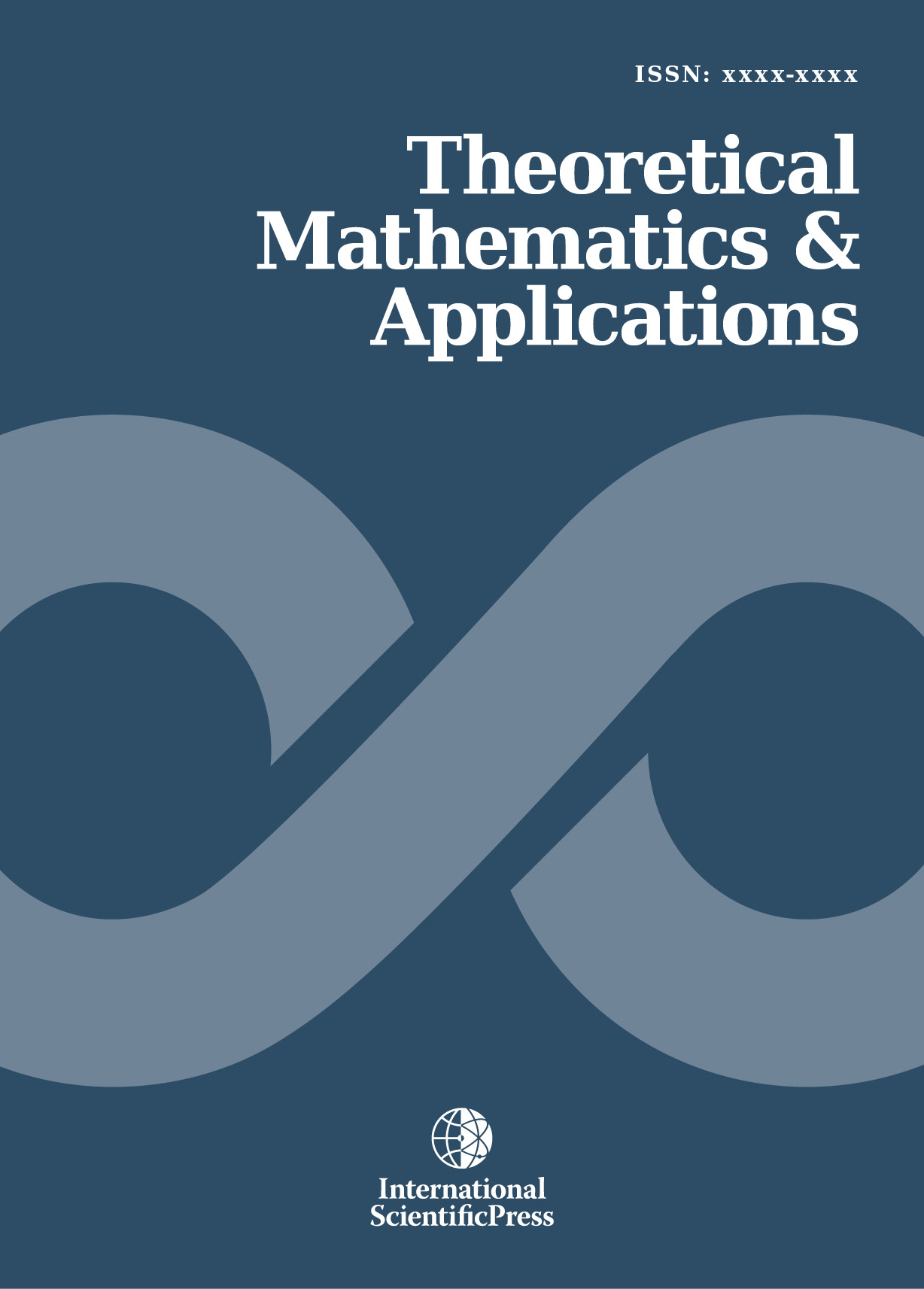# Theoretical Mathematics & Applications

#### On Admissible Mordell Equations and Hall conjecture

•[ Download ]
• Times downloaded: 1732
•                                                   Abstract

We introduce the notion of an admissible Mordell equation and establish some basic results concerning properties of its integral solutions. A non class-number and complete parametrization, with the most minimal set of conditions, for generating all the integral solutions of admissible Mordell equations was then proved. An effective and efficient algorithm for computing the integral solutions of admissible Mordell equations was also established from a proof of the Hall conjecture. One of our major results concerns the upper-bound for the number of integral solutions of each of these equations which we show may be explicitly computed. The analysis of these results leads us to the problem of deriving a general expression for the number of integral solutions of the Mordell equations. This problem is completely solved.ISSN: 1792-9687 (Print)
1792-9709 (Online)